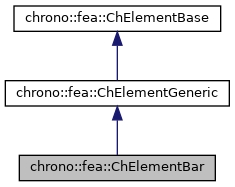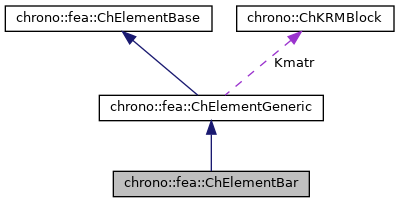chrono::fea::ChElementBar Class Reference

## Description

Simple finite element with two nodes and a bar that connects them.

No bending and torsion stiffness, just like a bar with two spherical joints. In practical terms, this element works a bit like the class ChElementSpring, but also adds mass along the element, hence point-like mass in the two nodes is not needed.

#include <ChElementBar.h>

Inheritance diagram for chrono::fea::ChElementBar:[legend]
Collaboration diagram for chrono::fea::ChElementBar:[legend]

## Public Member Functions

virtual int GetNnodes () override
Gets the number of nodes used by this element.

virtual int GetNdofs () override
Gets the number of coordinates in the field used by the referenced nodes. More...

virtual int GetNodeNdofs (int n) override
Get the number of coordinates from the n-th node that are used by this element. More...

virtual std::shared_ptr< ChNodeFEAbaseGetNodeN (int n) override
Access the nth node.

virtual void SetNodes (std::shared_ptr< ChNodeFEAxyz > nodeA, std::shared_ptr< ChNodeFEAxyz > nodeB)

virtual void GetStateBlock (ChVectorDynamic<> &mD) override
Fills the D vector with the current field values at the nodes of the element, with proper ordering. More...

virtual void ComputeKRMmatricesGlobal (ChMatrixRef H, double Kfactor, double Rfactor=0, double Mfactor=0) override
Sets H as the global stiffness matrix K, scaled by Kfactor. More...

virtual void ComputeInternalForces (ChVectorDynamic<> &Fi) override
Computes the internal forces (ex. More...

void SetBarArea (double ma)
Set the cross sectional area of the bar (m^2) (also changes stiffness keeping same E modulus)

double GetBarArea ()

void SetBarDensity (double md)
Set the density of the bar (kg/m^3)

double GetBarDensity ()

void SetBarYoungModulus (double mE)
Set the Young elastic modulus (N/m^2) (also sets stiffness)

double GetBarYoungModulus ()

void SetBarRaleyghDamping (double mr)
Set the Rayleigh damping ratio r (as in: R = r * K )

double GetBarRaleyghDamping ()

double GetMass ()
The full mass of the bar.

double GetRestLength ()
The rest length of the bar.

double GetCurrentLength ()
The current length of the bar (might be after deformation)

double GetStrain ()
Get the strain (epsilon), after deformation.

double GetStress ()
Get the elastic stress (sigma), after deformation.

virtual double GetCurrentForce ()
Get the current force transmitted along the bar direction, including the effect of the damper. More...Public Member Functions inherited from chrono::fea::ChElementGeneric
ChKblockGenericKstiffness ()
Access the proxy to stiffness, for sparse solver.

virtual void EleIntLoadResidual_F (ChVectorDynamic<> &R, const double c) override
(This is a default (a bit unoptimal) book keeping so that in children classes you can avoid implementing this EleIntLoadResidual_F function, unless you need faster code)

virtual void EleIntLoadResidual_Mv (ChVectorDynamic<> &R, const ChVectorDynamic<> &w, const double c) override
(This is a default (VERY UNOPTIMAL) book keeping so that in children classes you can avoid implementing this EleIntLoadResidual_Mv function, unless you need faster code.)

virtual void EleIntLoadResidual_F_gravity (ChVectorDynamic<> &R, const ChVector<> &G_acc, const double c) override
(This is a default (VERY UNOPTIMAL) book keeping so that in children classes you can avoid implementing this EleIntLoadResidual_F_gravity function, unless you need faster code. More...

virtual void ComputeGravityForces (ChVectorDynamic<> &Fg, const ChVector<> &G_acc) override
(This is the default implementation (POTENTIALLY INEFFICIENT) so that in children classes you can avoid implementing this ComputeGravityForces() function, unless you need faster code.) This fallback implementation uses a temp ChLoaderGravity that applies the load to elements only if they are inherited by ChLoadableUVW so it can use GetDensity() and Gauss quadrature.

virtual void ComputeMmatrixGlobal (ChMatrixRef M) override
Returns the global mass matrix. More...

virtual void InjectKRMmatrices (ChSystemDescriptor &mdescriptor) override
Tell to a system descriptor that there are item(s) of type ChKblock in this object (for further passing it to a solver)

virtual void KRMmatricesLoad (double Kfactor, double Rfactor, double Mfactor) override
Adds the current stiffness K and damping R and mass M matrices in encapsulated ChKblock item(s), if any. More...

virtual void VariablesFbLoadInternalForces (double factor=1.) override
Adds the internal forces, expressed as nodal forces, into the encapsulated ChVariables, in the 'fb' part: qf+=forces*factor (This is a default (a bit unoptimal) book keeping so that in children classes you can avoid implementing this VariablesFbLoadInternalForces function, unless you need faster code)

virtual void VariablesFbIncrementMq () override
Adds M*q (internal masses multiplied current 'qb') to Fb, ex. More...Public Member Functions inherited from chrono::fea::ChElementBase
virtual void ComputeNodalMass ()
Compute element's nodal masses.

virtual void Update ()
Update: this is called at least at each time step. More...

virtual void EleDoIntegration ()
This is optionally implemented if there is some internal state that requires integration.

## Additional Inherited MembersProtected Attributes inherited from chrono::fea::ChElementGeneric
ChKblockGeneric Kmatr

## ◆ ComputeInternalForces()

 void chrono::fea::ChElementBar::ComputeInternalForces ( ChVectorDynamic<> & Fi )
overridevirtual

Computes the internal forces (ex.

the actual position of nodes is not in relaxed reference position) and set values in the Fi vector.

Implements chrono::fea::ChElementBase.

## ◆ ComputeKRMmatricesGlobal()

 void chrono::fea::ChElementBar::ComputeKRMmatricesGlobal ( ChMatrixRef H, double Kfactor, double Rfactor = 0, double Mfactor = 0 )
overridevirtual

Sets H as the global stiffness matrix K, scaled by Kfactor.

Optionally, also superimposes global damping matrix R, scaled by Rfactor, and global mass matrix M multiplied by Mfactor. (For the spring matrix there is no need to corotate local matrices: we already know a closed form expression.)

Implements chrono::fea::ChElementBase.

## ◆ GetCurrentForce()

 double chrono::fea::ChElementBar::GetCurrentForce ( )
virtual

Get the current force transmitted along the bar direction, including the effect of the damper.

Positive if pulled. (N)

## ◆ GetNdofs()

 virtual int chrono::fea::ChElementBar::GetNdofs ( )
inlineoverridevirtual

Gets the number of coordinates in the field used by the referenced nodes.

This is for example the size (n.of rows/columns) of the local stiffness matrix.

Implements chrono::fea::ChElementBase.

## ◆ GetNodeNdofs()

 virtual int chrono::fea::ChElementBar::GetNodeNdofs ( int n )
inlineoverridevirtual

Get the number of coordinates from the n-th node that are used by this element.

Note that this may be different from the value returned by GetNodeN(n)->Get_ndof_w();

Implements chrono::fea::ChElementBase.

## ◆ GetStateBlock()

 void chrono::fea::ChElementBar::GetStateBlock ( ChVectorDynamic<> & mD )
overridevirtual

Fills the D vector with the current field values at the nodes of the element, with proper ordering.

If the D vector size is not this->GetNdofs(), it will be resized.

Implements chrono::fea::ChElementBase.

The documentation for this class was generated from the following files:
• /builds/uwsbel/chrono/src/chrono/fea/ChElementBar.h
• /builds/uwsbel/chrono/src/chrono/fea/ChElementBar.cpp